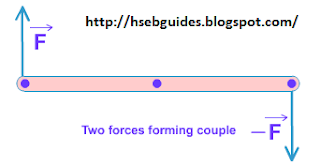# Couple Of Force

Definition
Two forces which are equal in magnitude but opposite in direction and not acting along the same line constitute a couple.Consider two equal and opposite forces F and -F acting oppositely along parallel lines on two points A and B as shown below:
Let and are their position vectors with respect to origin.
Magnitude of torque is given by:
= r F sinq
Where q is the angle between and.
rsinq is the perpendicular distance between the line of action of the two forces. Let it is denoted by d.
Thus, the magnitude of the torque of couple will be:
= Fd
Where d is called the moment arm of the couple.
Now
the magnitude of the couple = Magnitude of the forces forming couple X moment arm of couple